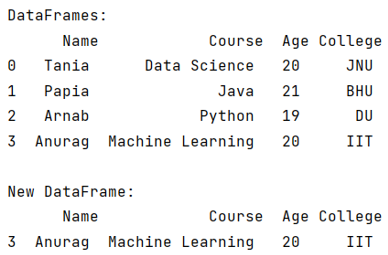# How to append only last row of a DataFrame to a new DataFrame?

Given a Pandas DataFrame, we have to append only last row of it to a new DataFrame.
Submitted by Pranit Sharma, on June 07, 2022

Rows in pandas are the different cell (column) values that are aligned horizontally and also provide uniformity. Each row can have the same or different value. Rows are generally marked with the index number but in pandas, we can also assign index names according to the needs. In pandas, we can create, read, update and delete a column or row value.

## Problem statement

Given a Pandas DataFrame, we have to append only last row of it to a new DataFrame.

## Appending only last row of a DataFrame to a new DataFrame

To append only the last row of a DataFrame in a new DataFrame, use pandas.DataFrame.tail() method. This method is used when we want to access the DataFrame from last, it returns a DataFrame up to the last n rows where n is the values that will be passed inside the method as a parameter.

Syntax:

```DataFrame.tail(n='some_value')
```

To append only the last row of a DataFrame in a new DataFrame, we will first access the last row of the old DataFrame with the help of pandas.DataFrame.tail() method and then we will assign this row to the new DataFrame.

Note

To work with pandas, we need to import pandas package first, below is the syntax:

```import pandas as pd
```

Let us understand with the help of an example,

## Python program to append only last row of a DataFrame to a new DataFrame

```# Importing pandas package
import pandas as pd

#  Creating a dictionary
d= {
'Name':['Tania','Papia','Arnab','Anurag'],
'Course':['Data Science','Java','Python','Machine Learning'],
'Age':[20,21,19,20],
'College':['JNU','BHU','DU','IIT']
}

# Creating a DataFrame
df = pd.DataFrame(d)

# Display DataFrames
print("DataFrames:\n",df,"\n")

# Creating a new DataFrame
new_df = pd.DataFrame

# Appending the last row of old DataFrame
# into new DataFrame
new_df = df.tail(1)

# Display new DataFrame
print("New DataFrame:\n",new_df)
```

### Output

The output of the above program is: